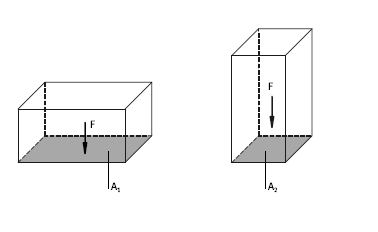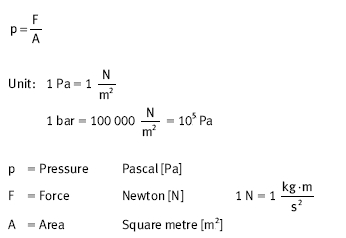﻿ Hydraulic Force, Area – Hydraulic Schematic Troubleshooting

## Hydraulic Force, Area

Every body exerts a specific pressure p on its base. The value of this pressure is dependent on the force due to weight F of the body and on the size of the area A on which the force due to weight acts.The diagram shows two bodies with different bases (AT1T and AT2T). Where the bodies have identical mass, the same force due to weight (F) acts on the base. However, the pressure is different owing to the different sizes of base. Where the force due to weight is identical, a higher pressure is produced in the case of a small base than in the case of a larger base (“pencil” or “concentrated” effect). This is expressed by the following formula: